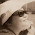## Tuesday, 16 February 2016

### Introduction to Algebra... Crystal Ball Gazing...Could you UNCOVER THE MYSTERY behind the crystal ball?
(in the Algebraic way!)

1.(10x+y)-(x+y)=9x
1)eg:89 8 is in the tens place so the digit (x10)
y-y=0
10x-x=9x since they are like terms
8 will be x / 9 will be y
8X10+9=89 89-(8+9)=72 ------multiple of 9
2)eg:21
2 is x / 1 is y
2X10+1=21 21-(2+1)=18--------multiple of 9

1.Peng Kiang:
Well done. The working is correct and pretty clear.
One way to improve is the presentation so that the reader could follow the description more closely.
I've re-written your working as follows:

Let x and y be 2 variables such that
x represents the TEN place digit and y represents the UNIT place digit.

E.g. 89 = 10x + 9

Now, (10x + y) - (x + y)
We simplify to get: 10x + y - x - y
that results 9x only.

The result, 9x is a multiple of 9.
That explains why the value we obtained from the 2-step calculation is always a multiple of 9

Test with one example: 89
Step 1: 8X10 + 9 = 89
Step 2: 89 - (8+9) = 72 -------multiple of 9

Test with another example: 21
21 = where x = 2 and y = 1
Step 1: 2X10 + 1 = 21
Step 2: 21 - (2+1) = 18 -------multiple of 9

2.The symbols are randomised, the only thing staying the same is the symbols of the multiples of nine. Since the answer will always be a multiple of nine, the program will be right unless a calculation error is made. In programming terms it would be
randomise symbols, keep multiples of nine the same symbol----wait for click on the crystal ball----show the symbol for multiples of nine----infinite loop.

1.Jonathan

You are right about the programming part, to make the display look 'randomised' yet there is an order within it.
Clear description of how it works in the algorithm.

Note: only a member of this blog may post a comment.